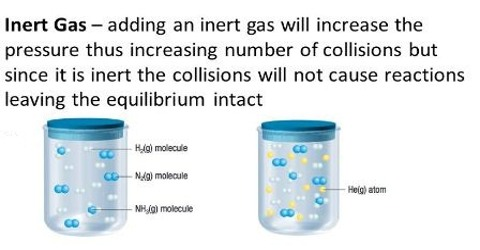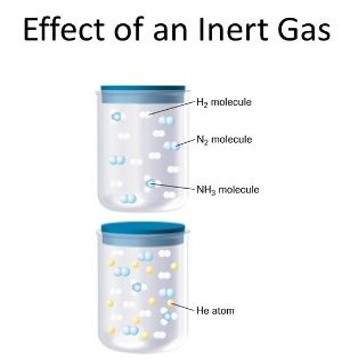# Effect of Adding an Inert Gas

Effect of adding an inert gas

If an inert (non-reacting) gas is added to a system of gaseous equilibrium at constant volume (the total pressure will increase), the equilibrium is not affected. This follows from Dalton’s law of partial pressures, because according to this law the presence of a foreign inert gas in a closed space does not influence the partial pressure exerted by the reacting substances. This is true whether the reaction involves a change in the number of molecules or not. If, however, the pressure is kept constant while the inert gas is added to the system at equilibrium, (there is a consequent increase in volume), the equilibrium is displaced, according to the Le Chatelier principle, in cases of reactions where KP is dependent on pressure. If KP is independent of pressure, as in the decomposition of hydrogen iodide, there is no effect.When an inert gas is added to the system in equilibrium at constant pressure, then the entire volume will raise. Hence, the number of moles per unit volume of diverse reactants and products will reduce. Hence, the equilibrium will shift towards the direction in which there is increase in number of moles of gases.

Consider the following reaction in equilibrium:

N2 (g) + 3H2 (g) ↔ 2NH3 (g)

The addition of an inert gas at constant pressure to the above reaction will shift the equilibrium towards the forward direction because the number of moles of products is more than the number of moles of the reactants.

Increasing the pressure by adding an inert gas at constant volume has no outcome. This is for the reason that what matters for the equilibrium are the partial pressures of the reactants and products. Adding an inert element to a system at constant volume will change the entire pressure but not the partial pressures of the compounds of interest.